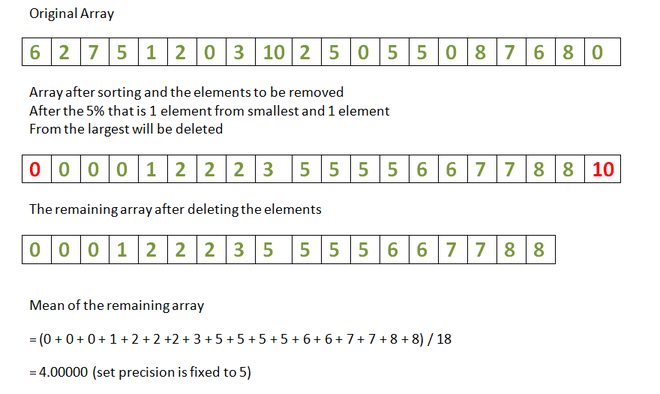# Mean of given array after removal of K percent of smallest and largest array elements

• Last Updated : 12 May, 2021

Given an array arr[] and an integer K, the task is to remove K % percent array elements from the smallest and largest array elements and calculate the mean of the remaining array.

Examples:

Attention reader! Don’t stop learning now. Get hold of all the important DSA concepts with the DSA Self Paced Course at a student-friendly price and become industry ready.  To complete your preparation from learning a language to DS Algo and many more,  please refer Complete Interview Preparation Course.

In case you wish to attend live classes with experts, please refer DSA Live Classes for Working Professionals and Competitive Programming Live for Students.

Input: arr[] = {6, 2, 7, 5, 1, 2, 0, 3, 10, 2, 5, 0, 5, 5, 0, 8, 7, 6, 8, 0}, K = 5
Output: 4.00000
Explanation:
There are 20 elements in the array. Therefore, 5% of 20 is 1. Therefore, 1 of the smallest elements (i.e. 0) is removed and 1 element of the largest elements (i.e. 0) is removed. Therefore, mean of the remaining array is 18.Input: arr[] = {6, 0, 7, 0, 7, 5, 7, 8, 3, 4, 0, 7, 8, 1, 6, 8, 1, 1, 2, 4}, K = 10
Output: 4.31250

Approach:

1. Sort the array arr[].
2. Find the size of the array.
3. Calculate the K-th percent of the size of the array.
4. Now, add the elements present in the indices K% to (N – 1) – K%.
5. Finally, find the mean of those elements.

Below is the implementation of the above approach:

## C++14

 `// C++ program for the above approach``#include ``using` `namespace` `std;` `// Function to calculate the mean``// of a given array after removal``// of Kth percent of smallest and``// largest array elements``void` `meanOfRemainingElements(``int` `arr[],``                             ``int` `N, ``int` `K)``{``    ``// Sort the array``    ``sort(arr, arr + N);` `    ``// Find the K-th percent``    ``// of the array size``    ``int` `kthPercent = (N * K) / 100;``    ``float` `sum = 0;` `    ``// Traverse the array``    ``for` `(``int` `i = 0; i < N; i++)` `        ``// Skip the first K-th``        ``// percent & last K-th``        ``// percent array elements``        ``if` `(i >= kthPercent && i < (N - kthPercent))``            ``sum += arr[i];` `    ``// Mean of the rest of elements``    ``float` `mean = sum``                 ``/ (N - 2 * kthPercent);` `    ``// Print mean upto 5 decimal places``    ``cout << fixed << setprecision(5) << mean << endl;``}` `// Driver Code``int` `main()``{` `    ``int` `arr[] = { 6, 2, 7, 5, 1, 2, 0, 3, 10, 2,``                  ``5, 0, 5, 5, 0, 8, 7, 6, 8, 0 };``    ``int` `arr_size = ``sizeof``(arr) / ``sizeof``(arr);``    ``int` `K = 5;` `    ``meanOfRemainingElements(arr, arr_size, K);` `    ``return` `0;``}`

## Java

 `// Java program for the above approach``import` `java.util.*;``public` `class` `GFG``{` `  ``// Function to calculate the mean``  ``// of a given array after removal``  ``// of Kth percent of smallest and``  ``// largest array elements``  ``static` `void` `meanOfRemainingElements(``int``[] arr, ``int` `N,``                                      ``int` `K)``  ``{` `    ``// Sort the array``    ``Arrays.sort(arr);` `    ``// Find the K-th percent``    ``// of the array size``    ``int` `kthPercent = (N * K) / ``100``;``    ``float` `sum = 0f;` `    ``// Traverse the array``    ``for` `(``int` `i = ``0``; i < N; i++)` `      ``// Skip the first K-th``      ``// percent & last K-th``      ``// percent array elements``      ``if` `(i >= kthPercent && i < (N - kthPercent))``        ``sum += arr[i];` `    ``// Mean of the rest of elements``    ``float` `mean = (sum / (N - ``2` `* kthPercent));` `    ``// Print mean upto 5 decimal places``    ``System.out.format(``"%.5f"``, mean);``  ``}`  `  ``// Driver Code``  ``public` `static` `void` `main(String args[])``  ``{``    ``int``[] arr = { ``6``, ``2``, ``7``, ``5``, ``1``, ``2``, ``0``, ``3``, ``10``, ``2``,``                 ``5``, ``0``, ``5``, ``5``, ``0``, ``8``, ``7``, ``6``, ``8``,  ``0` `};``    ``int` `arr_size = arr.length;``    ``int` `K = ``5``;` `    ``meanOfRemainingElements(arr, arr_size, K);``  ``}``}` `// This code is contributed by jana_sayantan.`

## Python3

 `# Python program for the above approach` `# Function to calculate the mean``# of a given array after removal``# of Kth percent of smallest and``# largest array elements``def` `meanOfRemainingElements(arr, N, K):``  ` `    ``# Sort the array``    ``arr.sort()` `    ``# Find the K-th percent``    ``# of the array size``    ``kthPercent ``=` `(N ``*` `K) ``/` `100``    ``sum` `=` `0` `    ``# Traverse the array``    ``for` `i ``in` `range``(N):` `        ``# Skip the first K-th``        ``# percent & last K-th``        ``# percent array elements``        ``if` `(i >``=` `kthPercent ``and` `i < (N ``-` `kthPercent)):``            ``sum` `+``=` `arr[i]` `    ``# Mean of the rest of elements``    ``mean ``=` `sum``/` `(N ``-` `2` `*` `kthPercent)` `    ``# Print mean upto 5 decimal places``    ``print``( ``'%.5f'``%``mean)` `# Driver Code``arr ``=` `[ ``6``, ``2``, ``7``, ``5``, ``1``, ``2``, ``0``, ``3``, ``10``, ``2``, ``5``, ``0``, ``5``, ``5``, ``0``, ``8``, ``7``, ``6``, ``8``, ``0` `]``arr_size ``=` `len``(arr)``K ``=` `5` `meanOfRemainingElements(arr, arr_size, K)` `# This code is contributed by rohitsingh07052.`

## C#

 `// C# program for the above approach``using` `System;` `class` `GFG {` `    ``// Function to calculate the mean``    ``// of a given array after removal``    ``// of Kth percent of smallest and``    ``// largest array elements``    ``static` `void` `meanOfRemainingElements(``int``[] arr, ``int` `N,``                                        ``int` `K)``    ``{``      ` `        ``// Sort the array``        ``Array.Sort(arr);` `        ``// Find the K-th percent``        ``// of the array size``        ``int` `kthPercent = (N * K) / 100;``        ``float` `sum = 0f;` `        ``// Traverse the array``        ``for` `(``int` `i = 0; i < N; i++)` `            ``// Skip the first K-th``            ``// percent & last K-th``            ``// percent array elements``            ``if` `(i >= kthPercent && i < (N - kthPercent))``                ``sum += arr[i];` `        ``// Mean of the rest of elements``        ``float` `mean = (sum / (N - 2 * kthPercent));` `        ``// Print mean upto 5 decimal places``        ``Console.WriteLine(Math.Round(mean,5));``    ``}` `    ``// Driver Code``    ``public` `static` `void` `Main()``    ``{``        ``int``[] arr = { 6, 2, 7, 5, 1, 2, 0, 3, 10, 2,``                      ``5, 0, 5, 5, 0, 8, 7, 6, 8,  0 };``        ``int` `arr_size = arr.Length;``        ``int` `K = 5;` `        ``meanOfRemainingElements(arr, arr_size, K);``    ``}``}` `// This code is contributed by chitranayal.`

## Javascript

 ``

Output:
`4.00000`

Time Complexity: O(N * logN)
Auxiliary Space: O(N)

My Personal Notes arrow_drop_up